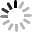Up to 5 files, each 10M size is supported. OK
News Get a QuoteHome - News - What is the formula for turning rpm？
Categories

# What is the formula for turning rpm？

September 6, 2023

# What is the formula for turning rpm?

Revolutions per minute (rpm) is a widely used unit of measurement in various fields, including physics, engineering, and mechanics. It represents the number of complete rotations an object makes in one minute. Understanding how to convert and calculate rpm is essential for many applications. In this article, we will explore the formula for turning rpm, generate three questions related to it, and solve them to draw conclusive answers.

## Question 1: How do you convert rpm to radians per second?

To convert rpm to radians per second, we need to understand the relationship between rotations and radians in a given time frame. Since one complete rotation is equivalent to 2π radians, we can use this conversion factor to calculate the desired quantity.

Let's solve an example to demonstrate the conversion: Suppose we have an object spinning at 1200 rpm. To convert this value to radians per second:

## Question 2: How can we calculate the linear speed from rpm and the radius of rotation?

When an object rotates, it generates linear motion at its outermost surface. To determine the linear speed in terms of rpm and the radius of rotation, we can utilize the concept of circumference.

Formula: Linear speed = (rpm × 2π × radius) / 60

Let's solve an example to illustrate this calculation: Consider a wheel rotating at 500 rpm with a radius of 0.5 meters. To find the linear speed:

Linear speed = (500 × 2π × 0.5) / 60 = 26.18 m/s (approx.)

## Question 3: How can we calculate the time taken to complete a certain number of revolutions at a given rpm?

Sometimes, we may need to find the time it takes for an object to complete a specific number of revolutions based on the given rpm. To determine this time duration, we can use the following formula:

Formula: Time = (number of revolutions) / (rpm / 60)

Let's work on an example to provide a clear understanding: Suppose a wheel rotates at 300 rpm and we want to find the time it takes to complete 6 revolutions:

Time = 6 / (300 / 60) = 0.12 minutes (or 7.2 seconds)

## Conclusion:

In conclusion, we have explored three questions related to rpm calculations. We learned how to convert rpm to radians per second, calculate linear speed using rpm and radius, as well as determine the time taken for a given number of revolutions at a specific rpm. These formulas and calculations are fundamental for various fields where the concept of rotation and speed is important. By understanding and applying these formulas, we can make accurate measurements and predictions in real-world scenarios.